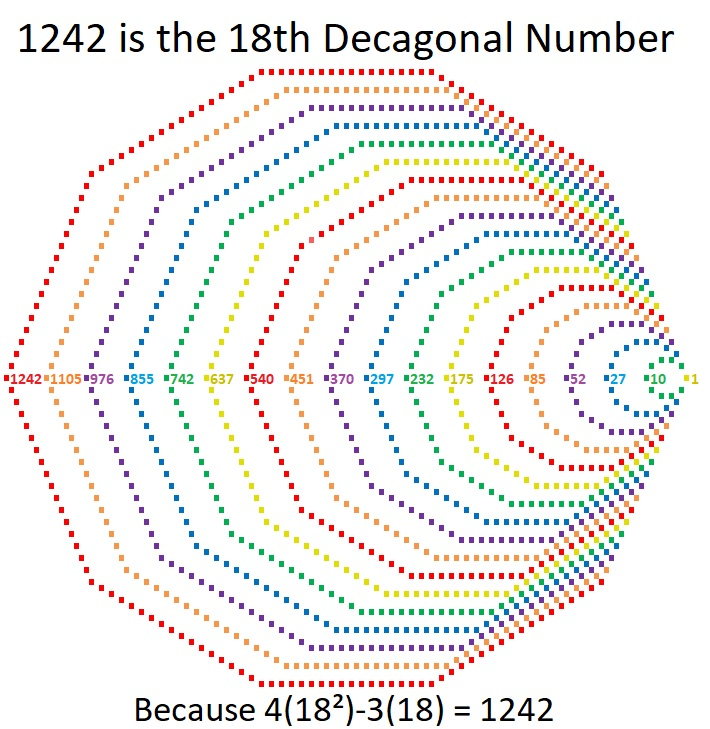# 1242 is a Decagonal Number

If you had 1242 tiny little squares you could arrange them into a decagon, just as I did for the graphic below.18 is a factor of 1242. Since 18(4·18-3) = 18(69) = 1242, it is the 18th decagonal number.

Here are a few more facts about the number 1242:

• 1242 is a composite number.
• Prime factorization: 1242 = 2 × 3 × 3 × 3 × 23, which can be written 1242 = 2 × 3³ × 23
• The exponents in the prime factorization are 1, 3, and 1. Adding one to each and multiplying we get (1 + 1)(3 + 1)(1 + 1) = 2 × 2 × 2 = 16. Therefore 1242 has exactly 16 factors.
• Factors of 1242: 1, 2, 3, 6, 9, 18, 23, 27, 46, 54, 69, 138, 207, 414, 621, 1242
• Factor pairs: 1242 = 1 × 1242, 2 × 621, 3 × 414, 6 × 207, 9 × 138, 18 × 69, 23 × 54, or 27 × 46
• Taking the factor pair with the largest square number factor, we get √1242 = (√9)(√138) = 3√138 ≈ 35.242021242 is the sum of consecutive prime numbers two different ways:
It is the sum of the eighteen prime numbers from 31 to 107, and
it is also the sum of the sixteen prime numbers from 43 to 109.

This site uses Akismet to reduce spam. Learn how your comment data is processed.